### 15.4 Flow Chart of CompressibleFlowSolver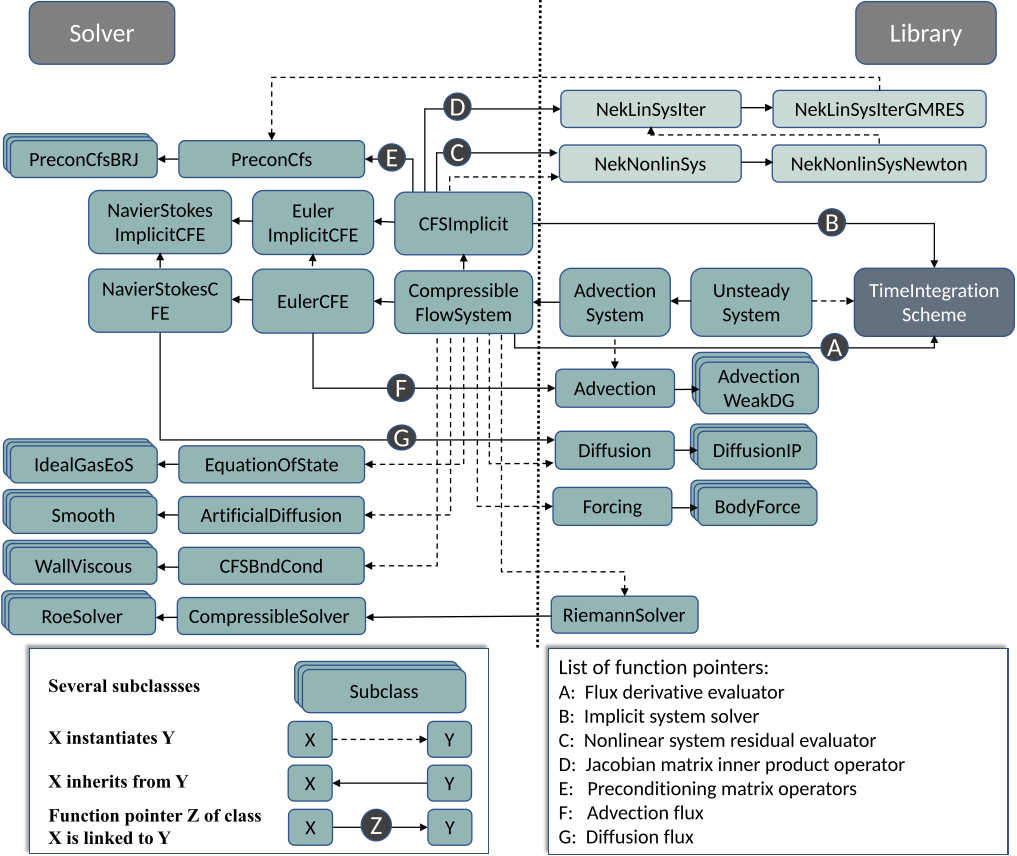Figure 15.2 Class structure of the explicit and implicit solvers. The equation system classes (` EulerCFE` or ` NavierStokesCFE`) contain access to the main functionalities of the solver, such as time integration, and the solution fields. They make use of numerical methods from the libraries, such as ` AdvectionWeakDG`, and equations system related functions, such as the advection flux (` F`) and diffusion flux (` G`), to form the spatial discretization operator ` A`. The spatial operator ` A` and the time integration method form the explicit solver using the method of lines. For the implicit solver, additional classes like the Newton solver (` NekNonlinSysNewton`), GMRES solver (` NekLinSysIterGMRES`) and linear algebra solver of preconditioners (e.g. ` PrecondCfsBRJ`) are instantiated. Together with operators related to the nonlinear system (` C`), the Jacobian matrix (` D`) and preconditioning matrix (` E`), an implicit system solver (` B`) is constructed, which is linked to the implicit time integration scheme to form the implicit solver.

The main procedures for building a solver are briefly introduced using the explicit solver of the NS equations as an example. Besides the main solver class (` CompressibleFlowSolver`) which controls the solving procedure, an equation system class is needed. The equation system classes (` EulerCFE` or ` NavierStokesCFE`) are instantiated and initialized dynamically based on user inputs using a factory method pattern [?], which is also extensively used for the dynamic object creation of classes in the solver. The equation system class inherits instantiations of classes related to geometry information, solution approximation, time integration and others from equations system classes in the libraries such as ` UnsteadySystem` in ` SolverUtils`. Thus the main functionality of the equation system class is to offer equation system related functions and to form spatial discretization operators using numerical methods from the libraries such as, ` AdvectionWeakDG`. Fig. 15.2 illustrates the class structure of the explicit and implicit solvers. The equation of state (` EquationOfState`), boundary conditions (` CFSBndCond`), Riemann flux (` RiemmanSolver`), shock capturing method (` ArtificialDiffusion`), forcing term (` Forcing`), advection flux (function pointer ` F`) and diffusion flux (function pointer ` G`) are instantiated or implemented in the equation system class. Specific numerical schemes like the weak DG scheme for advection terms (` AdvectionWeakDG`) and the interior penalty method for diffusion terms (` DiffusionIP`) are instantiated to calculate ∇⋅F and ∇⋅G in Eq. (15.1) provided with all these equation system related functions (` F` and ` G`). Finally the spatial discretization operator Lδ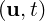is linked to the time integration class using the pointer-to-function ` A` and the explicit solver is complete.

The main simulation flowchart of the implicit solver is given in Tab. 15.2, which also shows the flow between different classes.

Table 15.2 Nassi–Shneiderman diagram of the implicit solver with the corresponding class names. Brown: library class ; cyan: solver class.

Initialization
Time step loop n
Output and finalization
 in `CompressibleFlowSolver` in `UnsteadySystem` Runge-Kutta loop m = 1,,M in `TimeIntegrationScheme` Calculate source term S m in `TimeIntegrationScheme` Netwon iteration l in `NewtonSolver` Calculate residual N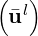in `CFSImplicit` GMRES iteration k in `NekLinSysIterGMRES` Calculate search vector qk in `NekLinSysIterGMRES` BRJ iteration j = 1,,J in `PrecondBRJ` Calculate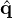j =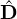-1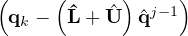in `CFSImplicit` Calculate ∂N∕∂u ⋅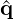J in `CFSImplicit` Calculate linear system residual in `NekLinSysIterGMRES` Calculate ul+1 by linear combination of qk in `NekLinSysIterGMRES` Calculate un+1 in `TimeIntegrationScheme` in `CompressibleFlowSolver`